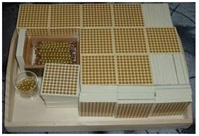ACTIVITY:

## Montessori Dynamic Multiplication

MATERIALS:A set of large number cards

Three sets of small number cards

A felt lined tray for each child

2 tables – one for the beads (bank)

one for the cards

One floor mat (the operations mat)

A small changing pot

OBJECTIVES:

To teach the child about multiplication in the concrete form.

To show the child how to change when doing addition and multiplication since the rules are the same.

CONTROL OF ERROR:

NA

AGE: 3 – 6 years approx.
• The hundreds are brought down and counted and labelled as before.
• When all the beads are counted and all the corresponding quantities in number cards have been placed below them, the cards are then overlapped.
• The large overlapped cards are then placed below the small number cards, which have remained on the right hand side of the mat.
• The Montessori Directress then explains to the children that this time we added up three numbers that were exactly the same quantity. When we add three numbers that are the same, then we are multiplying the number three times. This is called multiplication.
• Re-state the operation saying, “each child collected 1141 beads and we added all three numbers (or we can say we multiplied it three times) and now we have a larger number, 1423.
• Remind the child how we changed the tens when we had more than 10 tens.
• End the work cycle.
• Sorry, the comment form is closed at this time.